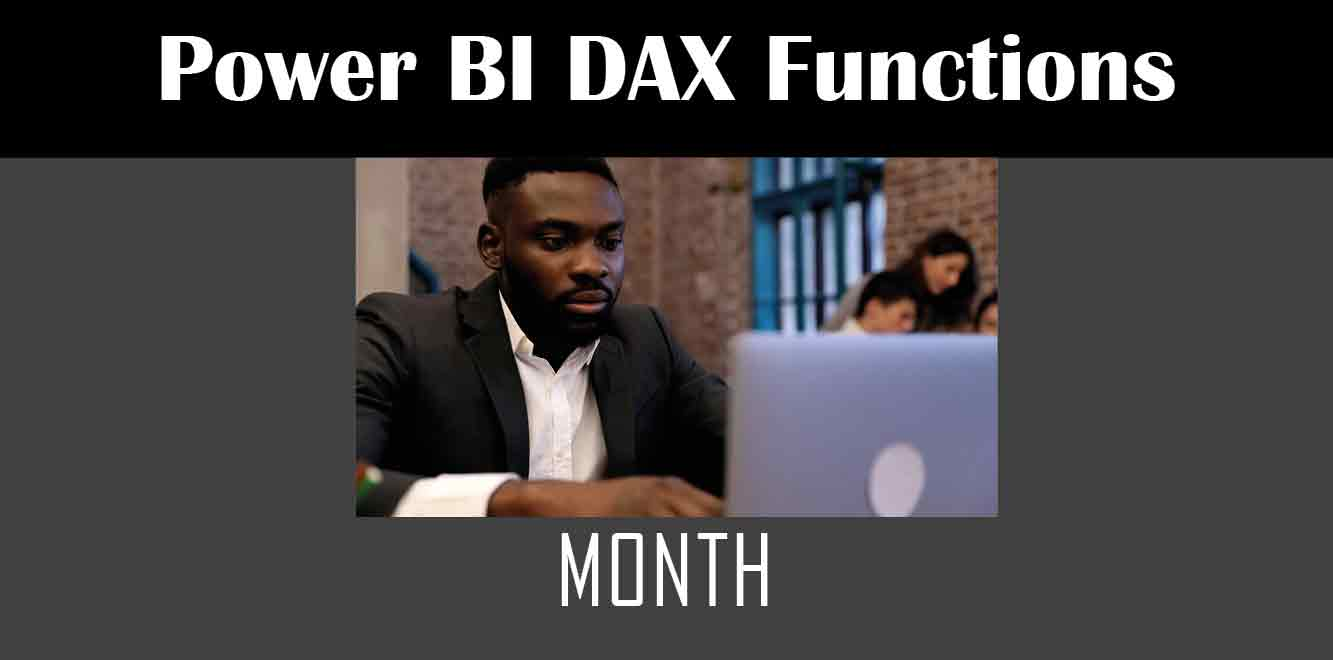# Month

## Y## Syntax

The syntax for the Power Fx Month function is as follows:

Month(Date)

``` This function takes a single argument, which is a date. The function returns the month component of the date as a number between 1 and 12. Examples Let's take a look at some examples to better understand how the Power Fx Month function works: Example 1 Suppose we have a date value of "1/15/2022." To extract the month component from this date, we can use the following formula: ```

Month(“1/15/2022”)

``` The above formula will return a value of 1, which corresponds to the month of January. Example 2 Suppose we have a date value of "6/12/2022." To extract the month component from this date, we can use the following formula: ```

Month(“6/12/2022”)

``` The above formula will return a value of 6, which corresponds to the month of June. Usage The Power Fx Month function can be used in a variety of scenarios, such as: Filtering Suppose we have a gallery control that displays a list of items with a date field. We can use the Power Fx Month function to filter the items based on a particular month. For example, to filter the items for the month of January, we can use the following formula: ```

Filter(Items, Month(Date)=1)

``` Grouping Suppose we have a collection of data that contains a date field. We can use the Power Fx Month function to group the data by month. For example, to group the data by month and get a count of items for each month, we can use the following formula: ```

GroupBy(Items, “Month”, “Month(Date)”, “Count”)

``` Calculations Suppose we have two date fields and we want to calculate the difference between the months. We can use the Power Fx Month function to extract the month component from each date and then subtract them. For example, to calculate the difference between the months of two dates, we can use the following formula: ```

Month(Date1)-Month(Date2)

``` In this article, we discussed how to use the Power Fx Month function in Power Apps. We learned about the syntax and examples of the function and also explored its usage in filtering, grouping, and calculations. The Power Fx Month function is a powerful tool that can help you manipulate and analyze date data in Power Apps. Power Apps Training Courses by G Com Solutions (0800 998 9248)1-Week Intensive Power Apps Training Course £4,975.00 Select optionsContinue Loading DonePower Apps Introduction (2 Days) £1,990.00 Select optionsContinue Loading DonePower Apps Intermediate (1 Day) £995.00 Select optionsContinue Loading DonePower Apps Advanced (2 Days) £1,990.00 Select optionsContinue Loading Done ```
``` ```
``` ```
``` ```
``` ```
``` ```